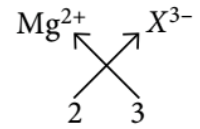Periodic Trends
Question

# Magnesium reacts with an element (X) to form an ionic compound. If the ground state electronic configuration of (X) is $1{s}^{2}2{s}^{2}2{p}^{3},$ the simplest formula for this compound is

Easy
Solution

## Electronic configuration of X is $1{s}^{2},2{s}^{2},2{p}^{3}.$ It is a 15th group element.it requires three electrons to attain octet. So, valency of X will be 3 . Magnesium ion $={\mathrm{Mg}}^{2+}$Formula : ${\mathrm{Mg}}_{3}{X}_{2}$

Get Instant Solutions